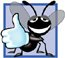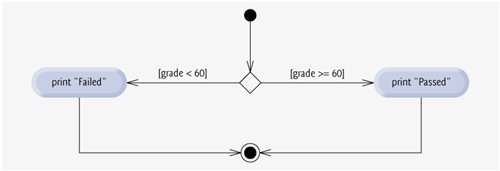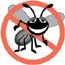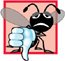# if...else Double-Selection Statement

The if single-selection statement performs an indicated action only when the condition is TRue; otherwise the action is skipped. The if...else double-selection statement allows the programmer to specify an action to perform when the condition is true and a different action to perform when the condition is false. For example, the pseudocode statement

```If student's grade is greater than or equal to 60
Print "Passed"
Else
Print "Failed"
```

prints "Passed" if the student's grade is greater than or equal to 60, but prints "Failed" if the student's grade is less than 60. In either case, after printing occurs, the next pseudocode statement in sequence is "performed."

The preceding pseudocode If...Else statement can be written in C++ as

```if ( grade >= 60 )
cout << "Passed";
else
cout << "Failed";
```

Note that the body of the else is also indented. Whatever indentation convention you choose should be applied consistently throughout your programs. It is difficult to read programs that do not obey uniform spacing conventions.

Good Programming Practice 4.2Indent both body statements of an if...else statement.

Good Programming Practice 4.3If there are several levels of indentation, each level should be indented the same additional amount of space.

Figure 4.5 illustrates the flow of control in the if...else statement. Once again, note that (besides the initial state, transition arrows and final state) the only other symbols in the activity diagram represent action states and decisions. We continue to emphasize this action/decision model of computing. Imagine again a deep bin of empty UML activity diagrams of double-selection statementsas many as the programmer might need to stack and nest with the activity diagrams of other control statements to form a structured implementation of an algorithm. The programmer fills in the action states and decision symbols with action expressions and guard conditions appropriate to the algorithm.

Figure 4.5. if...else double-selection statement activity diagram.Conditional Operator (?:)

C++ provides the conditional operator (?:), which is closely related to the if...else statement. The conditional operator is C++'s only ternary operatorit takes three operands. The operands, together with the conditional operator, form a conditional expression. The first operand is a condition, the second operand is the value for the entire conditional expression if the condition is TRue and the third operand is the value for the entire conditional expression if the condition is false. For example, the output statement

```cout << ( grade >= 60 ? "Passed" : "Failed" );
```

contains a conditional expression, grade >= 60 ? "Passed" : "Failed", that evaluates to the string "Passed" if the condition grade >= 60 is TRue, but evaluates to the string "Failed" if the condition is false. Thus, the statement with the conditional operator performs essentially the same as the preceding if...else statement. As we will see, the precedence of the conditional operator is low, so the parentheses in the preceding expression are required.

Error-Prevention Tip 4.1To avoid precedence problems (and for clarity), place conditional expressions (that appear in larger expressions) in parentheses.

The values in a conditional expression also can be actions to execute. For example, the following conditional expression also prints "Passed" or "Failed":

```grade >= 60 ? cout << "Passed" : cout << "Failed";
```

The preceding conditional expression is read, "If grade is greater than or equal to 60, then cout << "Passed"; otherwise, cout << "Failed"." This, too, is comparable to the preceding if...else statement. Conditional expressions can appear in some program locations where if...else statements cannot.

Nested if...else Statements

Nested if...else statements test for multiple cases by placing if...else selection statements inside other if...else selection statements. For example, the following pseudocode if...else statement prints A for exam grades greater than or equal to 90, B for grades in the range 80 to 89, C for grades in the range 70 to 79, D for grades in the range 60 to 69 and F for all other grades:

If student's grade is greater than or equal to 90
Print "A"
Else
If student's grade is greater than or equal to 80
Print "B"
Else
If student's grade is greater than or equal to 70
Print "C"
Else
If student's grade is greater than or equal to 60
Print "D"
Else
Print "F"

This pseudocode can be written in C++ as

```if ( studentGrade >= 90 ) // 90 and above gets "A"
cout << "A";
else
if ( studentGrade >= 80 ) // 80-89 gets "B"
cout << "B";
else
if ( studentGrade >= 70 ) // 70-79 gets "C"
cout << "C";
else
if ( studentGrade >= 60 ) // 60-69 gets "D"
cout << "D";
else // less than 60 gets "F"
cout << "F";
```

If studentGrade is greater than or equal to 90, the first four conditions will be true, but only the cout statement after the first test will execute. After that cout executes, the program skips the else-part of the "outermost" if...else statement. Most C++ programmers prefer to write the preceding if...else statement as

```if ( studentGrade >= 90 ) // 90 and above gets "A"
cout << "A";
else if ( studentGrade >= 80 ) // 80-89 gets "B"
cout << "B";
else if ( studentGrade >= 70 ) // 70-79 gets "C"
cout << "C";
else if ( studentGrade >= 60 ) // 60-69 gets "D"
cout << "D";
else // less than 60 gets "F"
cout << "F";
```

The two forms are identical except for the spacing and indentation, which the compiler ignores. The latter form is popular because it avoids deep indentation of the code to the right. Such indentation often leaves little room on a line, forcing lines to be split and decreasing program readability.

Performance Tip 4.1A nested if...else statement can perform much faster than a series of single-selection if statements because of the possibility of early exit after one of the conditions is satisfied.

Performance Tip 4.2In a nested if...else statement, test the conditions that are more likely to be true at the beginning of the nested if...else statement. This will enable the nested if...else statement to run faster and exit earlier than testing infrequently occurring cases first.

Dangling-else Problem

The C++ compiler always associates an else with the immediately preceding if unless told to do otherwise by the placement of braces ({ and }). This behavior can lead to what is referred to as the dangling-else problem. For example,

```if ( x > 5 )
if ( y > 5 )
cout << "x and y are > 5";
else
cout << "x is <= 5";
```

appears to indicate that if x is greater than 5, the nested if statement determines whether y is also greater than 5. If so, "x and y are > 5" is output. Otherwise, it appears that if x is not greater than 5, the else part of the if...else outputs "x is <= 5".

Beware! This nested if...else statement does not execute as it appears. The compiler actually interprets the statement as

```if ( x > 5  )
if ( y > 5 )
cout << "x and y are > 5";
else
cout << "x is <= 5";
```

in which the body of the first if is a nested if...else. The outer if statement tests whether x is greater than 5. If so, execution continues by testing whether y is also greater than 5. If the second condition is true, the proper string"x and y are > 5"is displayed. However, if the second condition is false, the string "x is <= 5" is displayed, even though we know that x is greater than 5.

To force the nested if...else statement to execute as it was originally intended, we must write it as follows:

```if ( x > 5 )
{
if ( y > 5 )
cout << "x and y are > 5";
}
else
cout << "x is <= 5";
```

The braces ({}) indicate to the compiler that the second if statement is in the body of the first if and that the else is associated with the first if. Exercise 4.23 and Exercise 4.24 further investigate the dangling-else problem.

Blocks

The if selection statement normally expects only one statement in its body. Similarly, the if and else parts of an if...else statement each expect only one body statement. To include several statements in the body of an if or in either part of an if...else, enclose the statements in braces ({ and }). A set of statements contained within a pair of braces is called a compound statement or a block. We use the term "block" from this point forward.

Software Engineering Observation 4.2A block can be placed anywhere in a program that a single statement can be placed.

The following example includes a block in the else part of an if...else statement.

```if ( studentGrade >= 60 )
cout << "Passed.
";
else
{
cout << "Failed.
";
cout << "You must take this course again.
";
}
```

In this case, if studentGrade is less than 60, the program executes both statements in the body of the else and prints

Failed.

You must take this course again.

Notice the braces surrounding the two statements in the else clause. These braces are important. Without the braces, the statement

```cout << "You must take this course again.
";
```

would be outside the body of the else part of the if and would execute regardless of whether the grade is less than 60. This is an example of a logic error.

Common Programming Error 4.3Forgetting one or both of the braces that delimit a block can lead to syntax errors or logic errors in a program.

Good Programming Practice 4.4Always putting the braces in an if...else statement (or any control statement) helps prevent their accidental omission, especially when adding statements to an if or else clause at a later time. To avoid omitting one or both of the braces, some programmers prefer to type the beginning and ending braces of blocks even before typing the individual statements within the braces.

Just as a block can be placed anywhere a single statement can be placed, it is also possible to have no statement at allcalled a null statement (or an empty statement). The null statement is represented by placing a semicolon (;) where a statement would normally be.

Common Programming Error 4.4Placing a semicolon after the condition in an if statement leads to a logic error in single-selection if statements and a syntax error in double-selection if...else statements (when the if part contains an actual body statement).C++ How to Program (5th Edition)
ISBN: 0131857576
EAN: 2147483647
Year: 2004
Pages: 627